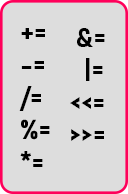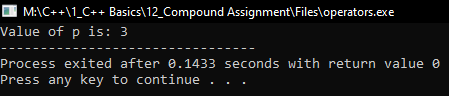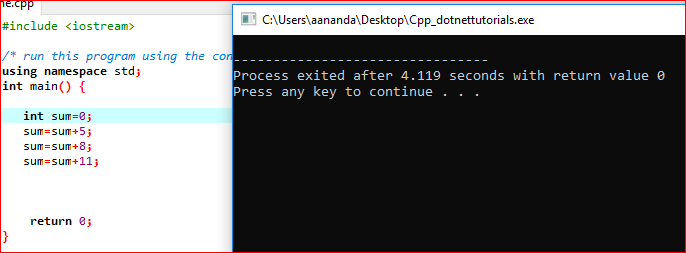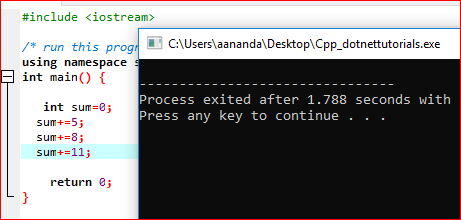# Compound Assignment Operator in C++

## Compound Assignment Operator in C++ with Examples

In this article, I am going to discuss Compound Assignment Operator in C++ with Examples. Please read our previous article where we discussed Programming Exercises in C++. We have already created a few simple programs using formulas and expressions where we used use arithmetic operators. We have a lot of other operators to learn so slowly we’ll be learning about them. Now, we will learn about compound assignment operators.

##### Compound Assignment Operator in C++

Compound Assignment is there not only for arithmetic operators but it is there for other operators also. However, we will discuss compound assignment arithmetic operators later whenever we discuss those operators at that time, I will discuss the compound operators also.

These are related to arithmetic as well as other operators also. So let us understand what these are and when they are useful. We have listed the compound assignment operators:You can see that the operators coming before the assignment. Usually, the expression or all the operators will be after the assignment but here it is coming before the assignment.

##### When it is useful?

When it is useful let us see. For that I have taken one example here,

int x = 3, y = 15, z = 21;

int sum = 3;

We have some variables x, y, and the sum which are having some values. Now the first thing is we want to perform the addition between sum and a and store the result in sum itself. So let us see how we can do that.

We can write it as sum = sum + a;

This statement means we add sum and a, and store the result in sum. Now the sum becomes 6. These types of statements are commonly used in programming. Now we will see the same statement can be written using the Compound Assignment Operator. Let us see this.

sum = sum + a;

In the above expression, the sum is used on the right-hand side of the assignment as well as the left-hand side of the assignment. So, the same thing can be written as

sum += a;

So instead of writing sum 2 times, we can write it as above. Now, this is easily readable. For a beginner, it is not readable but when you are writing C++ programming you get used to it. Writing the above statement by using a compound assignment operator is faster than the previous method. Internally compiler will make it faster. So, this statement is faster.

##### Compound Assignment Operator Example:

Now we take another example:
int x = 3, y = 4, z = 2;
int p = 1;

In some program we have to perform many operations on a single variable, so at that time we can use compound assignment.
p *= x; — (i)
p *= y; — (ii)
p -= x + y + z; — (iii)

Here In the 1st statement, we are performing multiplication between p and x and then store the result in p itself. Here value of p will 1 * 3 = 3. When we execute the first statement, the value of p will 3.

Now the 2nd statement, we are performing multiplication between p and y and store results in p. But here the value of p is 3 as evaluated from 1st statement. Now the value of p will be 3 * 4 = 12. So, at this point, the p value will be 12.

In the 3rd statement, we are performing subtraction between p and the result of the addition of x, y, and z. And store that in p. Here p = 12. So, at execution, it will be 12 – (3+4+2) = 3. Now p = 3

Here we modified ‘p’ with multiple values. So, in such situations, we use this type of operator. That is a compound assignment. It can be done for subtraction, multiplication, division, and all the other operators like bitwise operators. We will look at them in the coming articles. Let’s see the code part:

##### Compound Assignment Operator Code in C++ Language:
```#include <iostream>
using namespace std;
int main ()
{
int x = 3, y = 4, z = 2;
int p = 1;

p *= x;
p *= y;
p -= x + y + z;

cout << "Value of p is: " << p;
return 0;
}
```
###### Output:##### Why Compound Assignment Operator?

Compound assignment operator usually does the same thing that existing operators are doing but it gives more efficiency in compile time. To explain the compound assignment Operator let us take an example: I have a variable I want to repeat add another no to this variable. Currently, without a compound assignment operator, I am following this method. I am writing a pseudocode

int sum=0;
Sum=sum+5;
Sum=sum+8;
Sum=sum+11; //here every time you are adding sum with other values and storing back it in sum only.

The Variable sum is evaluated in each instruction, which consumes more compile-time hence inefficient. To overcome this problem and also to increase program readability compound assignment operators or shorthand operators come into the picture. Now the same pseudo-code can be written as

int sum=0;
Sum+=5;
Sum+=8;
Sum+=11;
Let’s see how efficient it is by comparing the compile time for both.

##### Without compound assignment operator

Without shorthand operator/compound assignment operator Compile-time is 4.119 seconds##### With compound assignment operator

With shorthand operator/compound assignment operator Compile-time is only 1.788 seconds.Note: Assignment and Compound Assignment Operators have the least precedence when compare to other arithmetic operators.

In the next article, I am going to discuss Increment Decrement Operator in C++ with Examples. Here, in this article, I try to explain Compound Assignment Operator in C++ with Examples and I hope you enjoy this Compound Assignment Operator in C++ with Examples article.

### 1 thought on “Compound Assignment Operator in C++”

1.you are one of the best teacher, I never saw this much nice explaination